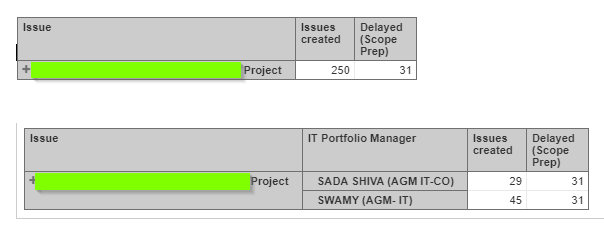# Aggregation of a Calculated Measure at Dimension Level

Hi, Everyone,

I have to calculate the number of instances(issue count), there is a delay based on the planned date and the actual date.

For this purpose, I have created a calculated measure to calculate the delay.

"[Measures].[Variation(Scope Prep)]" = DateDiffWorkdays([Measures].[Issue Scope Preparation Planned End Date], [Measures].[Issue Scope Preparation Actual End Date])

Using this calculated measure, I have created another calculated measure to get the count and get is aggregated at the dimension level

"[Measures].[Delayed (Scope Prep)]" = NonZero( Sum(
Filter(
Descendants([Issue].CurrentHierarchyMember, [Issue].[Issue]),
[Measures].[Variation(Scope Prep)]>0
),
[Measures].[Variation(Scope Prep)].Count
)
)

I have got the aggregation at the project level correctly. However, the measure is displaying the same value at each row, even if I add another dimension to the rows, which is the wrong count.Please guide me on how to get values aggregated at dimension level (similar to that of the measure Issues Created)

Hey @Sudheer,
First of all - welcome to our eazyBI community!

Second - about your question - you need to use some measure in your formula that is not an Issue property. Also, use Count() instead of Sum(). You can change your formula accordingly to this.

``````NonZero(
Count(
Filter(
Descendants([Issue].CurrentHierarchyMember, [Issue].[Issue]),
[Measures].[Issues created]> 0 AND
[Measures].[Variation(Scope Prep)]>0
)
)
)
``````

Kind regards,
Gerda // support@eazybi.com

Thanks, Gerda.

I have got it sorted out with a similar solution.

I have a follow-up query, I have created this custom field, for which returns another calculated field [Variation(Scope Prep)] at each issue-level and sum of the calculated fields at the project level.

However, when a new dimension is added to the report in addition to the current dimension - Issue, the values are not getting aggregated at the combination of the two dimensions(Say Time). So I have added a case statement and use different filter conditions to address this issue.

My issue is I have some 20 dimensions and this will give me a huge number of combinations. Is there a better way of handling this type of scenarios?

Hey @Sudheer,
could you share the formula that you are using and some print screen of your report just to be sure we are on the same page?
As calculation that I shared should be split by different dimensions (in case of Time, it would use Issue created date as this date is used in this formula).

best,
Gerda

Hi, @Gerda,

My base calculated measure is based on the difference between two date fields.

`**[Measures].[Dev Days (Resolved)]** = DateDiffWorkdays([Measures].[Issue Start date],[Measures].[Issue resolution date])`

I need to calculate the average of this field based on the dimensions selected in the report.

I have started with three dimensions

• Issue Type
• Time
• IT Portfolio Manager (default dimension)

Here’s the code I am using

``````Case WHEN [Issue Type].CurrentMember.level.Name <> 'Issue Type' THEN -- When Issue Type dimension is not selected
Avg(
Filter(
Descendants([Issue].CurrentHierarchyMember,[Issue].[Issue]),
[Measures].[Issue IT Portfolio Manager] = [IT Portfolio Manager].CurrentMember.Name
AND  DateInPeriod( [Measures].[Issue resolution date], [Time].CurrentHierarchyMember)
),
[Measures].[Dev Days (Resolved)]
)

WHEN [Time].CurrentHierarchyMember IS [Time].CurrentHierarchy.DefaultMember THEN -- When Time Period dimension is not selected

Avg(
Filter(
Descendants([Issue].CurrentHierarchyMember,[Issue].[Issue]),
[Measures].[Issue IT Portfolio Manager] = [IT Portfolio Manager].CurrentMember.Name
AND  [Measures].[Issue type] = [Issue Type].CurrentMember.Name
),
[Measures].[Dev Days (Resolved)]
)

WHEN ([Issue Type].CurrentMember.level.Name <> 'Issue Type' AND
[Time].CurrentHierarchyMember IS [Time].CurrentHierarchy.DefaultMember) -- when both 'Issue Type' & Time dimensions are not selected.
THEN
Avg(
Filter(
Descendants([Issue].CurrentHierarchyMember,[Issue].[Issue]),
[Measures].[Issue IT Portfolio Manager] = [IT Portfolio Manager].CurrentMember.Name
),
[Measures].[Dev Days (Resolved)]
)

ELSE -- when all the three dimensions are selected

Avg(
Filter(
Descendants([Issue].CurrentHierarchyMember,[Issue].[Issue]),

[Measures].[Issue IT Portfolio Manager] = [IT Portfolio Manager].CurrentMember.Name
AND  [Measures].[Issue type] = [Issue Type].CurrentMember.Name
AND  DateInPeriod( [Measures].[Issue resolution date], [Time].CurrentHierarchyMember)
),
[Measures].[Dev Days (Resolved)]
)
END
``````

This works fine for the three dimensions. If I add one more dimension, I have add multiple case statements based on the combinations of the four dimensions and I have close to 20 dimensions, any 3/4 dimensions out of twenty can be used.

As your calculated measure is using Issue resolution date, you can filter issues by measure Issues resolved. Function Avg() returns the average value of a numeric expression evaluated over a set. Syntax of this function is `Avg(<Set>,<Numeric Expression>)` and if you use additional dimensions, it will take this context into account and adjust the set accordingly.

``````Avg(
Filter(
Descendants([Issue].CurrentHierarchyMember,[Issue].[Issue]),
[Measures].[Issues resolved] > 0 ),
[Measures].[Dev Days (Resolved)]
)
``````

best,
Gerda

1 Like

Thanks very much, Gerda. It worked like Magic!!!

1 Like

Excellent! it works like a charm.

1 Like